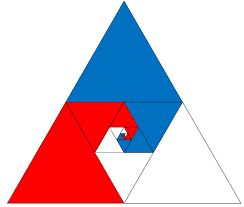Get inspired by the success stories of our students in IIT JAM MS, ISI  MStat, CMI MSc Data Science.  Learn More

# A Telescopic Sequence| ISI MStat 2018 PSB Problem 2This is a beautiful problem from ISI MStat 2018 problem 2, which uses the cute little ideas of telescopic sum and partial fractions.

## Problem

Let $\{x_{n}\}_{n \geq 1}$ be a sequence defined by $x_{1}=1$ and
$$x_{n+1}=\left(x_{n}^{3}+\frac{1}{n(n+1)(n+2)}\right)^{1 / 3}, \quad n \geq 1$$
Show that $\{x_{n}\}_{n \geq 1}$ converges and find its limit.

### Prerequisities

• Limit of a Sequence
• Partial Fraction $\frac{1}{n(n+1)(n+2)} = \frac1{n(n+1)(n+2)}=\frac12\cdot\frac1n-\frac1{n+1}+\frac12\cdot\frac1{n+2} = -\frac12\left(\underbrace{\frac1{n+1} -\frac1n}\right)+\frac12\left(\underbrace{\frac1{n+2}-\frac1{n+1}}\right)$
• Telescopic Sum $\sum_{i = 1}^{\infty} \left(\underbrace{\frac1{i+1} -\frac1i}\right) = \lim_{n \to \infty} \frac1n - 1 = -1$

## Solution

$x_{n+1} = (x_{n}^{3}+\frac{1}{n(n+1)(n+2)})^{1 / 3} \Rightarrow {x_{n+1}}^3 = x_{n}^{3}+\frac{1}{n(n+1)(n+2)}$

$\Rightarrow {x_{n+1}}^3 - x_{n}^{3} = \frac{1}{i(i+1)(i+2)}; x_1 = 1$.

$\Rightarrow \sum_{i = 1}^{n-1} {x_{i+1}}^3 - x_{i}^{3} = \sum_{i = 1}^{n-1} \frac{1}{n(n+1)(n+2)} ; x_1 = 1$.

$x_{n}^{3} - x_{1}^{3} = \sum_{i = 1}^{n-1} \frac{1}{i(i+1)(i+2)} = \sum_{i = 1}^{n-1} -\frac12\left(\underbrace{\frac1{i+1} -\frac1i}\right)+\frac12\left(\underbrace{\frac1{i+2}-\frac1{i+1}}\right)$

$\lim_{n \to \infty} (x_{n}^{3} - x_{1}^{3}) = \sum_{i = 1}^{\infty} \frac{1}{i(i+1)(i+2)} = \sum_{i = 1}^{\infty} -\frac12\left(\underbrace{\frac1{i+1} -\frac1i}\right)+\frac12\left(\underbrace{\frac1{i+2}-\frac1{i+1}}\right) = \frac14$

$\lim_{n \to \infty} x_{n}^{3} = \frac54 \Rightarrow \lim_{n \to \infty} x_{n} = ({\frac54})^\frac13$.

This is a beautiful problem from ISI MStat 2018 problem 2, which uses the cute little ideas of telescopic sum and partial fractions.

## Problem

Let $\{x_{n}\}_{n \geq 1}$ be a sequence defined by $x_{1}=1$ and
$$x_{n+1}=\left(x_{n}^{3}+\frac{1}{n(n+1)(n+2)}\right)^{1 / 3}, \quad n \geq 1$$
Show that $\{x_{n}\}_{n \geq 1}$ converges and find its limit.

### Prerequisities

• Limit of a Sequence
• Partial Fraction $\frac{1}{n(n+1)(n+2)} = \frac1{n(n+1)(n+2)}=\frac12\cdot\frac1n-\frac1{n+1}+\frac12\cdot\frac1{n+2} = -\frac12\left(\underbrace{\frac1{n+1} -\frac1n}\right)+\frac12\left(\underbrace{\frac1{n+2}-\frac1{n+1}}\right)$
• Telescopic Sum $\sum_{i = 1}^{\infty} \left(\underbrace{\frac1{i+1} -\frac1i}\right) = \lim_{n \to \infty} \frac1n - 1 = -1$

## Solution

$x_{n+1} = (x_{n}^{3}+\frac{1}{n(n+1)(n+2)})^{1 / 3} \Rightarrow {x_{n+1}}^3 = x_{n}^{3}+\frac{1}{n(n+1)(n+2)}$

$\Rightarrow {x_{n+1}}^3 - x_{n}^{3} = \frac{1}{i(i+1)(i+2)}; x_1 = 1$.

$\Rightarrow \sum_{i = 1}^{n-1} {x_{i+1}}^3 - x_{i}^{3} = \sum_{i = 1}^{n-1} \frac{1}{n(n+1)(n+2)} ; x_1 = 1$.

$x_{n}^{3} - x_{1}^{3} = \sum_{i = 1}^{n-1} \frac{1}{i(i+1)(i+2)} = \sum_{i = 1}^{n-1} -\frac12\left(\underbrace{\frac1{i+1} -\frac1i}\right)+\frac12\left(\underbrace{\frac1{i+2}-\frac1{i+1}}\right)$

$\lim_{n \to \infty} (x_{n}^{3} - x_{1}^{3}) = \sum_{i = 1}^{\infty} \frac{1}{i(i+1)(i+2)} = \sum_{i = 1}^{\infty} -\frac12\left(\underbrace{\frac1{i+1} -\frac1i}\right)+\frac12\left(\underbrace{\frac1{i+2}-\frac1{i+1}}\right) = \frac14$

$\lim_{n \to \infty} x_{n}^{3} = \frac54 \Rightarrow \lim_{n \to \infty} x_{n} = ({\frac54})^\frac13$.

This site uses Akismet to reduce spam. Learn how your comment data is processed.

### 2 comments on “A Telescopic Sequence| ISI MStat 2018 PSB Problem 2”

1.Bornali Roy says:

2.Aayushi Jain says:

please check if the ans is (7/4)^1/3 . I think there is minus missing in the 4th line .

### Knowledge Partner